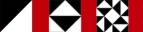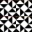Next Higher-Order Parsing 20

# 選択Alternation

• アトムは、本当はひとつではなく、3つのバリエーションがある

Atoms come in three varieties, not just one:

```        atom → NUMBER | VAR | function "(" expression ")"
```
• だからatomパーサにもこの3つをそれぞれ試してもらう必要がある

So we need the atom parser to try these three different things

• 失敗するのは、トークンがどれにもマッチしなかったときだけ

It fails only if the upcoming tokens match none of them

• こんな感じ

Something like this:

```        sub atom {
my \$in = shift;
my (\$result, \$out);
my \$alt3 = conc(lookfor("FUNC"),
lookfor("("), \$EXPRESSION,  lookfor(")"),
);

if (     (\$result, \$out) = lookfor("NUMBER")->(\$in)) {
return (\$result, \$out);
} elsif ((\$result, \$out) = lookfor("VAR")->(\$in)) {
return (\$result, \$out);
} elsif ((\$result, \$out) = \$alt3->(\$in)) {
return (\$result, \$out);
} else {
return;
}
}
```
• で、これもまた同じパターンになる関数が多いので

But again, many functions will follow this same pattern

• 小さなパーサをまとめる関数を書くことにしよう

So instead we'll write a function that assembles small parsers into big ones

• A, B,...というパーサ関数があるときに

Given parser functions A, B, etc.:

```        alt(A, B, ...)
```
• とすると A, B, ... と順に探してくれるようにするわけだ

Will return a parser function that looks for A or for B, etc.

 NextCopyright © 2007 M. J. Dominus